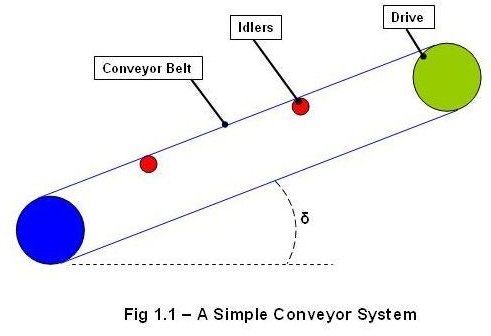# Conveyor Belt Calculations

The belt conveyor is used for conveying different materials from one location to another. The different components of a belt conveyor system typically are electric drives, pulleys, idlers, and a long belt. A simple conveyor system may look like below:## The basics of the Calculations of Conveyor Belt Design Parameters

• Belt tension: The belt of the conveyor always experience a tensile load due to the rotation of the electric drive, weight of the conveyed materials, and due to the idlers. The belt tension at steady state can be calculated as:

Tb = 1.37*f*L*g*[2*mi+ (2*mb + mm)*cos (δ)] + (H*g*mm)…….eqn.1.1

Where,

Tb is in Newton.

f = Coefficient of friction

L = Conveyor length in meters. Conveyor length is approximately half of the total belt length.

g = Acceleration due to gravity = 9.81 m/sec2

mi = Load due to the idlers in Kg/m.

mb = Load due to belt in Kg/m.

mm = Load due to the conveyed materials in Kg/m.

δ = Inclination angle of the conveyor in Degree.

H = vertical height of the conveyor in meters.

• Load due to idlers (mi): This can be calculated as below:

mi = (mass of a set of idlers) / (idlers spacing) ……………..eqn.1.2

Note: The article “Design an Idler for a Conveyor Belt Systemhas detailed explanation about how to calculate idlers spacing.

• Power at drive pulley: The power required at the drive pulley can be calculated from the belt tension value as below:

Pp = (Tb*V)/1000……………..eqn.1.3

Where,

Pp is in KW.

Tb = steady state belt tension in N.

v = belt speed in m/sec.

• Belt tension while starting the system: Initially during the start of the conveyor system, the tension in the belt will be much higher than the tension in steady state. The belt tension while starting can be calculated as:

Tbs =Tb*Ks………………..eqn.1.4

Where,

Tbs is in N.

Tb = the steady state belt tension in N.

Ks = the start-up factor

Pm = Pp/Kd………………eqn.1.5

Where,

Pm is in Kw.

Pp = the power at drive pulley in Kw

Kd = Drive efficiency.

• Acceleration : The acceleration of the conveyor belt can be calculated as:

A= (Tbs – Tb)/ [L*(2*mi + 2*mb+mm)]……………………eqn.1.6

Where,

A is in m/sec2

Tbs = the belt tension while starting in N.

Tb = the belt tension in steady state in N.

L = the length of the conveyor in meters.

mi = Load due to the idlers in Kg/m.

mb = Load due to belt in Kg/m.

mm = Load due to the conveyed materials in Kg/m.

• Belt breaking strength: This parameter decides the selection of the conveyor belt. The belt breaking strength can be calculated as:

Bs= (Cr*Pp)/ (Cv*V)…………..eqn.1.7

Where,

Bs is in Newton.

Cr = friction factor

Cv = Breaking strength loss factor

Pp = Power at drive pulley in Newton.

V = belt speed in m/sec.

## An Example of Conveyor Belt Calculations

Input data:

Conveyor capacity (Cc) = 1500 t/h = 416.67 Kg/sec

Belt speed (V) = 1.5 m/sec

Conveyor height (H) = 20 m

Conveyor length (L) = 250 m

Mass of a set of idlers (m’i) = 20 Kg

Idler spacing (l’) = 1.2 m

Load due to belt (mb) = 25 Kg/m

Inclination angle of the conveyor (δ) = 5 0

Coefficient of friction (f) = 0.02

Start-up factor (Ks) = 1.5

Drive efficiency (Kd) = 0.9

Friction factor (Cr) = 15

Breaking strength loss factor (Cv) = 0.75

Calculation:

• First, we will use the eqn.1.2 for finding out the load due to idlers:

mi = (20/1.2) = 16.67 Kg/m

• We will use the eqn.1.1 for finding out the belt tension in steady state:

Tb = 1.37*0.02*250*9.81*[16.67+ {2*25+ (416.67/1.5)}*cos (5)] + (20*9.81* (416.67/1.5)) = 77556.88 N.

• The belt tension while starting the system can be calculated by using the eqn.1.4:

Tbs = 1.5 * 77556.88 = 116335.32 N

• For calculating the power at drive pulley, we will use the eqn.1.3:

Pp = (77556.88*1.5)/ 1000 = 116.335 Kw

• We will use the eqn.1.5 estimate the size of the motor:

Pm = 116.35/0.9 = 129.261 Kw

• We will use the eqn.1.6 to find out the acceleration of the motor:

A = (116335.32 – 77556.88)/ [250*{(2*16.67) + (2*25) + (416.67/1.5)}]

= 0.429 m/sec2

• Lastly, we will use the eqn.1.7 to find out the belt breaking strength:

Bs = (15*116.35) / (0.75*1.5) = 1551.33 N/mm

This Bs value is used to select the conveyor belt from the manufacturer’s catalogue.

## Conclusion

The conveyor belt calculations methodology discussed in the article is to be used only for the guidance on calculating the initial conveyor design parameters; the final design must be validated by using the FEA or other similar tools before building the prototype.

Reference

• Dunlop – Conveyor belt technique design and calculation
• Bridgestone Conveyor Belt Design Manual